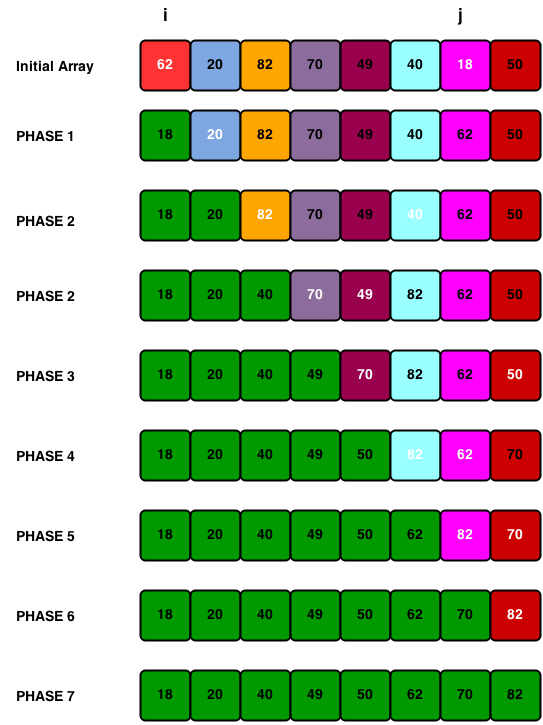Sorting allows us an efficient arrangement of elements within a given data structure. It is a way in which the elements are organized systematically.

In this tutorial we are learning about a most frequently used Selection Sort.

## Selection Sort

The selection sort in Java is very simple and effective to use is it in a larger array of elements in the array list. It will not require no more than n-1 interchanges.

Example :

x is an array of size n, which stored in memory. The selection sort algorithm first selects the smallest element in the array x and place it at array position 0; then it selects the next smallest elementin the array x and place it at array position 1. It continues this procedure until it places the biggest element in the last position of the array. here the array passed n-1 times.

Efficiency is O(n2) for n data items.

Example :

[java]

import java.util.ArrayList;

public final class SelectionSort {

private ArrayList<Integer> array = null;

public ArrayList<Integer> getArray() {
return array;
}

public void setArray(ArrayList<Integer> array) {
this.array = array;
}

public SelectionSort() {
array = new ArrayList<Integer>();
}

public ArrayList<Integer> sort() {
int index = 0;
int value = 0;
int counter = 0;
int current_value = 0;

try {
System.out.println("The initial array is: " + getArray());
if (getArray() != null && !getArray().isEmpty()) {
while (counter < getArray().size()) {
boolean change = false;

value = getArray().get(counter);
current_value = value;
for (int position = counter; position < getArray().size(); position++) {
if (getArray().get(position) < current_value) {
change = true;
current_value = getArray().get(position);
index = getArray().indexOf(current_value);
}
}
if (change) {
// swapping takes place
getArray().set(counter, current_value);
getArray().set(index, value);
}

System.out.println("Phase : " + (counter + 1) + " " + getArray());
counter += 1;
}
} else {
System.out.println("Unable to sort array as there are no elements");
}
} catch (Exception e) {
e.printStackTrace();
}
return array;
}

public static void main(String[] args) {
try {
SelectionSort sort = new SelectionSort();
sort.sort();
} catch (Exception e) {
e.printStackTrace();
}
}
}

[/java]

Output :

```The initial array is: [62, 20, 82, 70, 49, 40, 18, 50]
Phase : 1 [18, 20, 82, 70, 49, 40, 62, 50]
Phase : 2 [18, 20, 82, 70, 49, 40, 62, 50]
Phase : 3 [18, 20, 40, 70, 49, 82, 62, 50]
Phase : 4 [18, 20, 40, 49, 70, 82, 62, 50]
Phase : 5 [18, 20, 40, 49, 50, 82, 62, 70]
Phase : 6 [18, 20, 40, 49, 50, 62, 82, 70]
Phase : 7 [18, 20, 40, 49, 50, 62, 70, 82]
Phase : 8 [18, 20, 40, 49, 50, 62, 70, 82]```

Phase 1: Find the location j of the smallest element in the array x , x, . . . . x[n-1],

and then interchange x[j] with x.

Phase 2: Leave the first element and find the location j of the smallest element in the

sub-array x, x, . . . . x[n-1], and then interchange x with x[j]. Then

x, x are sorted.

Phase 3: Leave the first two elements and find the location j of the smallest element in

the sub-array x, x, . . . . x[n-1], and then interchange x with x[j].

Then x, x, x are sorted.

Phase (n-1): Find the location j of the smaller of the elements x[n-2] and x[n-1], and

then interchange x[j] and x[n-2]. Then x, x, . . . . x[n-2] are sorted. Of

course, during this pass x[n-1] will be the biggest element and so the entire array is sorted.

Happy Learning 🙂